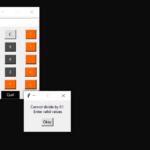Calculator App In Tkinter Python With Source Code

This Calculator App In Tkinter is a simple project developed using Python. The project contains the numbers, operators, and signs like on a normal calculator. Here, the user can just click on the numbers they want in the calculations. Hence, the user can ...

Type : Project

File Size : 1.6 KBSimilar Projects and Reports

Calculator App In Tkinter Python With Source Code

This Calculator App In Tkinter is a simple project developed using Python. The project contains the numbers, operators, and signs like on a normal calculator. Here, the user can just click on the numbers they want in the calculations. Hence, the user can ...

Calculator With Tkinter GUI In Python With Source Code

The Calculator With Tkinter GUI In Python is a simple project developed using Python. The project contains the numbers, operators, and signs like on a normal calculator. Here, the user can just click on the numbers they want in the calculations. Hence, th...

Calculator App In Python With Source Code

The Calculator App In Python is a simple project developed using Python. The project contains the numbers, operators, and signs like on a normal calculator. So, the user cannot enter the number themselves, they can just click on the numbers they want in t...

CALCULATOR IN PYTHON WITH SOURCE CODE

This Calculator project In Python is a simple project developed using Python. The project contains the numbers, operators, and sign like in normal calculator. So, the user can enter any number they want in the calculations. Hence, the user can use a simpl...

Tip Calculator In Python with Source Code

The Tip Calculator In Python is a simple project developed using Python. The project is for the user convenient, for calculating the tip. This project is an interesting project. The user can calculate the tip amount that they can get from a single bill pa...

Age Calculator In Python With Source Code

The Age Calculator In Python is a simple project developed using Python. The project is for the user convenient, to help them find out about their exact age in year, month and days. The project file contains a python script ( Age_Calculator_GUI.py). This ...